# Algebra

#### FUNCTIONS-1

The general form of  a quadratic function is f(x) = ax2 + bx + c, where a, b and c are real numbers and a ≠ 0. The degree of the expression is two. i.e. the highest exponent of the variable is two. Here a, b are the coefficients of x2 , x and c is the constant term.

Let us say f(x) =  x2 + 1. Now if we plot the curve of f(x), we get a parabola:

 Input =  x -2 -1 0 1 2 Output = f(x) 5 2 1 2 5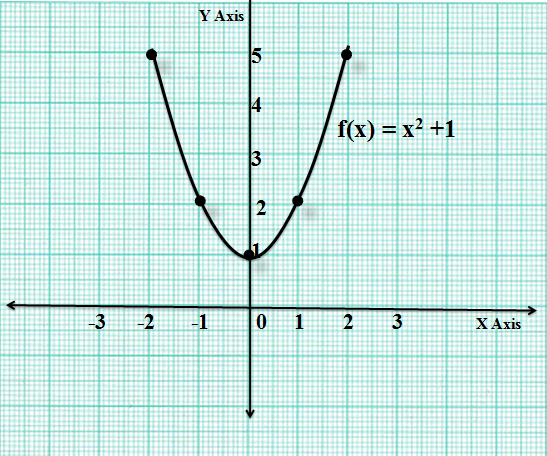Here the vertex of the parabola lies at the point (0,1). The point of minima is x = 0 and the minimum value of f(x) = 1.

If f(x) = ax2 + bx + c = 0 , then it is called a quadratic equation. This means that the value of the quadratic expression is zero. Here we find the values of x for which the quadratic assumes a value equal to zero. In other words we can say that the values of x for which the graph of f(x) cuts the  X axis.

Let f(x) = ax2 + bx + c = 0

The roots are those values of x for which the value of the quadratic expression becomes zero or  the values of x where the curve of f(x) cuts the X axis.

Let’s find the value of the roots.Nature of roots:

1. Real and Unequal Roots:4. Rational Roots:

If the quantity under the square root, i.e. the discriminant is a perfect square, then we can say that the roots are rational. So, if we want the roots to be rational then b2 -4ac must be a perfect square.

Sum and Product of the Roots of a Quadratic Equation:Remember: If a > the minimum value of f(x) occurs at x = -b/2a

If a < 0, then thGraph of a Quadratic Expression:

If we look at the curve of ax2 + bx + c, then definitely it is a parabola. Now if a > 0 , i.e. if the coefficient of x2 is positive, then it is open upwards and if a < 0, then the parabola is open downwards.

a). If the roots are real and unequal, then the curve of f(x) intersects the X axis at two points which are the roots say α and β. Part of the curve lies above the X axis and a part of it lies below the X axis. The curve looks like: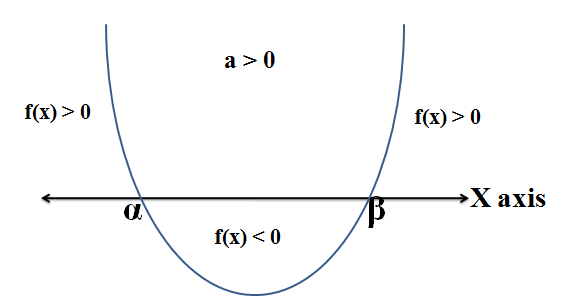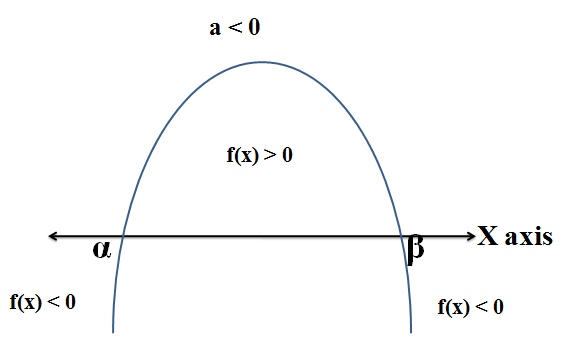So, we can say that when a > 0, and D > 0  then, f(x) > 0 when x < α and x > β

f(x) = 0 when x = α and β

f(x) < 0 when α < x < β

b). If the roots are real and equal

If D = 0, then the graph of f(x) touches the X axis at one point and at x = -b/2a which is the point of minima or maxima depending on the sign of a. So, the graph of f(x) looks like: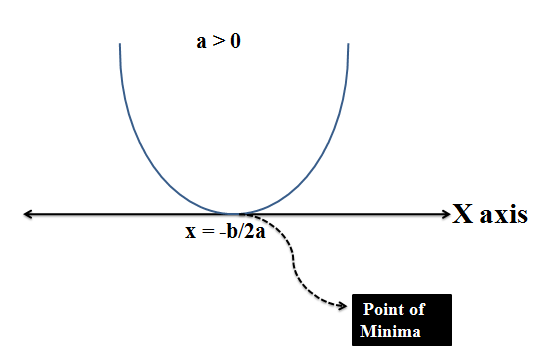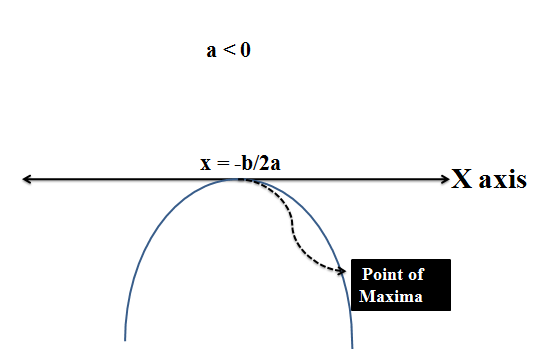So, if a >0 and D =0 , then  f(x) > 0 for all values of x except at x = -b/2a where f(x) = 0

a < 0 and D = 0, then f(x) < 0 for all values of x except at x = -b/2a where f(x) = 0

c). If roots are imaginary, then there exist no real roots. So, the entire curve is entirely above the X axis or below it depending on the sign of a. The minimum or the maximum value occurs at x = -b/2a .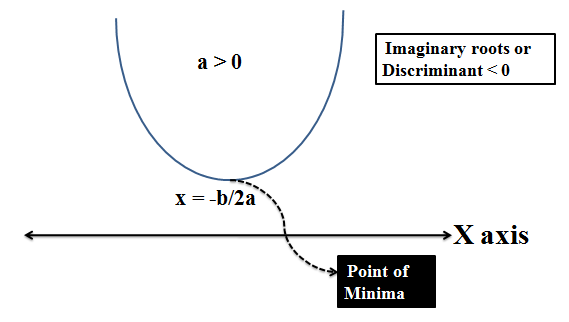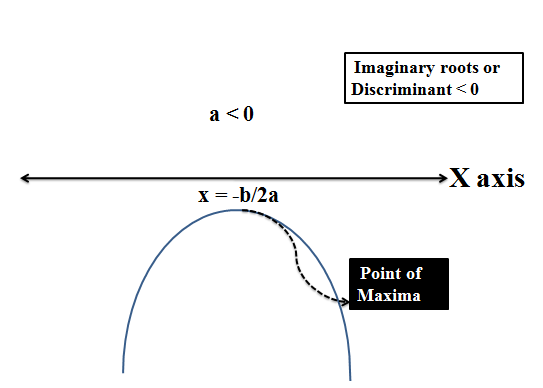So, if a > 0 and D < 0, then f(x) > 0   for all real values of x

If a < 0 and D < 0, then f(x) < 0 for all real values of x

Let us say we have a quadratic : x2 – 2x -3

This can be factorized as  x2 – 3x + x – 3    or x (x-3) + 1(x-3)

Or x2 – 2x – 3 =  (x-3)(x+1)

Here x = 3 and -1 are the roots of this quadratic and we have written the above quadratic in terms of its roots.

So if ax2 + bx + c  has two roots α and B, then we can write the quadratic in terms of its roots as:

ax2 + bx + c = a (x- α)(x-β)

Forming a New Quadratic Equation by changing the roots of a given quadratic equation:

1. A quadratic equation whose roots are ‘n’ more than the roots of a quadratic ax2 + bx + c is :

a(x-n)2 + b(x-n) + c = 0

2. A quadratic equation whose roots are ‘n’ less than the roots of a quadratic ax2 + bx + c is :

a(x+n)2 + b(x+n) + c = 0

3. A quadratic equation whose roots are ‘n’ times the roots of a quadratic ax2 + bx + c is :

a(x/n)2 + b(x/n) + c = 0

4. A quadratic equation whose roots are 1/n  times the roots of a quadratic ax2 + bx + c is :

a(nx)2 + b(nx) + c = 0

5. A quadratic equation whose roots are the  reciprocals of the roots of a quadratic ax2 + bx + c is :

a(1/x)2 + b(1/x) + c =0

6. A quadratic equation whose roots are negative of  the roots of a quadratic ax2 + bx + c is :

a(-x)2 + b(-x) + c = 0

7. A quadratic equation whose roots are the squares of  the roots of a quadratic ax2 + bx + c is :

a(√x)2 + b(√x) + c = 0

8. A quadratic equation whose roots are the cubes of the roots of a quadratic ax2 + bx + c is :

a(x1/3)2 + b(x1/3) + c = 0

Example 1: Find the maximum value of 4 - 5x - 4x2.

Solution:  Here f(x) = 4 – 5x – 4x2 , we know that the maxima occurs at x = -b/2a, here b = -5, a = -4

So maxima occurs at x = -b/2a = - (-5/-8) = -5/8

Maximum value of f(x) = 4 – 5(-5/8) – 4(-5/8)2 = 89/16

Example 2: If ax2 + bx - 15 = 0 does not have 2 distinct real roots, then find the maximum value of 3a + b?

Solution: Let f(x) = ax2 + bx – 15

Since f(x) = 0 does not have 2 distinct real roots, hence either
f(x)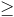0 for every x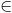R or f(x)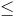0 for every xR.

Now since f(0) = -15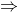f(x)0 for every xR.
In particular f (3)0.9a + 3b -150.3a + b5.
Hence the maximum value of 3a + b = 5.

Location of Roots/ Interval in which Roots Lie

In some problems we want the roots of the equation ax2 + bx + c = 0 to lie in a given Interval. For this we impose conditions on a, b and c.

Let f(x) = ax2 + bx + c.

Case 1: If both the roots are positive i.e. they lie in (0,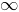),

Then the sum of the roots as well as the product of the roots must be positive

α+ β = -b/a >0     And αβ = c/a > 0   with  b2-4ac≥  0

Similarly, if both the roots are negative i.e. they lie in (–, 0) then the sum of

the roots will be negative and the product of the roots must be positive

i.e.  α+ β  = –b/a < 0    and  αβ = c/a >0  with b2 – 4ac ≥ 0.

Case 2 : Both the roots are greater than a number ‘k’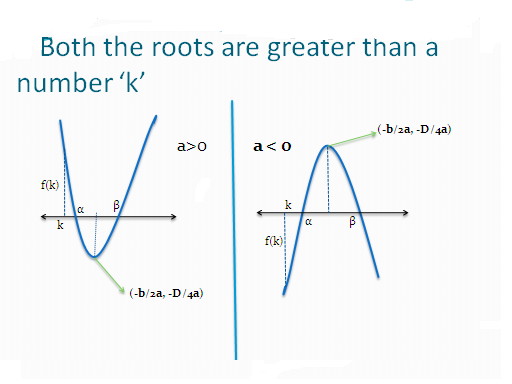In this case we observe that three conditions hold:

(i)  D ≥ 0 (ii) af (k) >0 (iii) k < (-b/2a) i.e. k should be less than the point of maxima or minima.

Both the roots are greater so the equation has real roots or equal roots. Hence D ≥ 0. Further we see that if a >0, then f(k) >0 and if a < 0 then f(k) is also <0. So the product of ‘a’ and f(k) is positive. As k is less than both the roots so k must be less than –b/2a.

Case3: If both the roots are less than ‘k’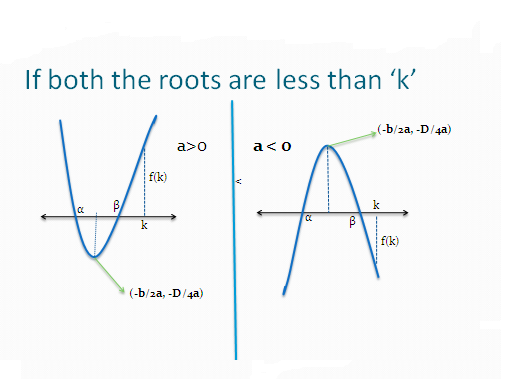In this case we see the following conditions hold:

(i) D ≥ 0 (ii) af (k) > 0 (iii)  k > (-b/2a)

Again the roots are real or equal so D ≥0. As we see that if a >0, then f(k) >0 and if a < 0 then f(k) is also <0. so the product a and f(k) is positive. As k is greater than both the roots so k > (-b/2a).

Case 4: If ‘k’ lies between the two roots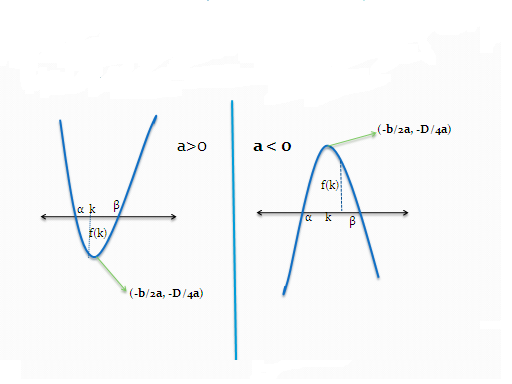In this case we have the following conditions:

i) D > 0 (ii) af (k) < 0

First of all k lies between the two roots. So D > 0 for roots to be real. Also when a < 0 then we see f (k) >o and when a is > 0 then f(k) is < 0. So product of ‘a’ and ‘f(k)’ is negative.

Case 5: if both the roots lie in the interval (k1, k2)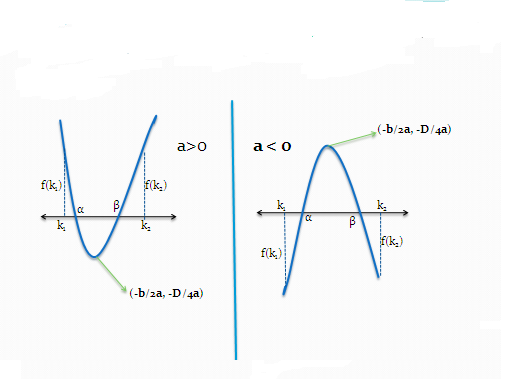Here the conditions that hold are:

1. D ≥ 0 (ii) af(k1) >0 (iii) af(k2) >0  (iv) k1 < (-b/2a) < k2 .

2. Here we are assuming that k1< k2.

Case6: Exactly one root lies in the interval (k1, k2)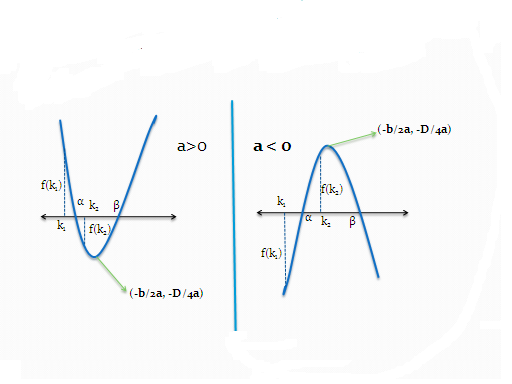In this case we have the following conditions:

(i)D > 0 (ii) f(k1). f(k2) < 0

As the equation has two roots. Discriminant must be positive .Also we observe that f(k1) and f(k2) are opposite in signs.

So their product is negative.

Case 7: If k1and k2 lie between the two roots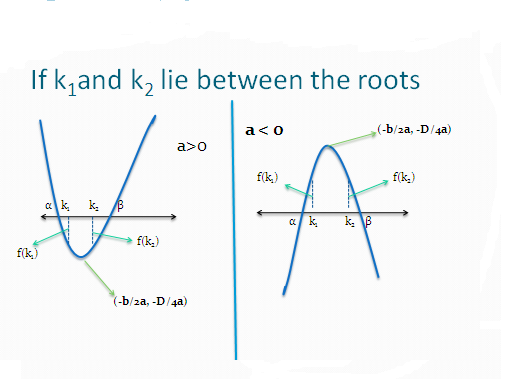The conditions that apply in this case are:

(i) D >0 (ii) a(f(k1) < 0 (iii)  a f(k2) < 0

Example 3: For all ‘x’, x2+ 2ax + (10-3a) >0, then the interval in which ‘a’ lies is :

A.  a < -5   B. -5 < a < 2     C. a > 5         D.  2 < a <5

Solution: Here a is positive and the expression is positive for all x.

So D < 0,

4a2-4(10-3a) < 0 or  a2+3a-10< 0

a2+5a-2a-10 < 0

(a+5)(a-2) < 0

or we can say -5 < a < 2

Hence option (b) is correct.

Example 4: Let a,b,c be real. If ax2+bx+c=0 has two real roots α and  β, where α < -1 and β > 1, then show that

1+ c/a+|b/a| < 0

Solution: Here the equation ax2+bx+c=0 has two real roots. So, discriminant is positive.a>0    a< 0?  -1 1   β    ?  -1   1  βWe see that when a is positive then f(-1) and f(1) both are negative

And when a is negative then f(-1) and f(1) both are positive.

af(-1) < 0 and     af(1) < 0

f(1)= a+b+c   and f(-1)= a-b+c

af (-1) = a (a+b+c) < 0 and      af (1)= a(a-b+c) < 0

Divide both side of inequation by a2

1+ (b/a) + (c/a) < 0 and 1-(b/a) +c/a< 0

Combining both these inequations we get

Or 1+|b/a|+c/a< 0

Example 5: Find two consecutive even natural numbers, sum of whose squares is 884

A. 20, 18                    B. 22, 24 C. 16, 18                                D. 20, 22

Solution: Let the two consecutive numbers be 2m and 2m +2,

Given that (2m)2 + (2m+2)2 = 884

4m2 + 4m2 + 4 + 8m = 884

Or 8m2 + 8m = 880    or m2 + m = 110

Or  solving we get  m = 10 and -11 , but m cannot be negative as m is a natural number

So, the numbers are 20 and 22

Option (D) is correct

Example 6: An aeroplane takes 2 hour less for the journey of 2400 Km, if its speed is increased by 100 Km/hr, from its usual speed . Find its usual speed.

A. 300 Km/hr

B. 400 Km/hr

C. 500 Km/hr

D. 600 Km/hr

Solution: Let the usual speed of the aeroplane be x km/hr

Time taken initially = 2400/x  {Time = Distance/speed}

New Speed = x+100

New time taken = 2400/(x+100)

But now the time taken is 2 hours less

So  2400/x  - 2400/(x+100) = 2

2400*100x(x+100)=2

Or 240000 = 2x2 + 200x

Or x2 + 100x = 120000

Or x2 + 400x – 300x -120000 = 0